Courses

# Principles of Torque Production Electrical Engineering (EE) Notes | EduRev

## Electrical Engineering (EE) : Principles of Torque Production Electrical Engineering (EE) Notes | EduRev

The document Principles of Torque Production Electrical Engineering (EE) Notes | EduRev is a part of the Electrical Engineering (EE) Course Electrical Machines for Electrical Engg..
All you need of Electrical Engineering (EE) at this link: Electrical Engineering (EE)

Principles of Torque Production

In the earlier section, we saw how a rotating flux is produced. Now let us consider a rotor, which is placed in this field. Let the rotor have a coil such that the coil sides are placed diametrically opposite each other. This is shown in the fig. 1. Since the flux generated by the stator rotates flux linked by this rotor coil also changes.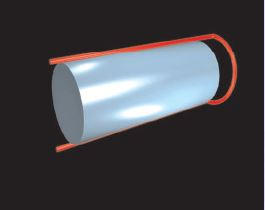Figure 1: A Coil on the rotor

Since the flux pattern is varying sinusoidally in space, as the flux waveform rotates, the flux linkage varies sinusoidally. The rate of variation of this flux linkage will then be equal to the speed of rotation of the air gap flux produced. This sinusoidal variation of the flux linkage produces a sinusoidal induced emf in the rotor coil. If the coil is short circuited, this induced emf will cause a current flow in the coil as per Lenz’s law.

Now imagine a second coil on the rotor whose axis is 120� away from the first. This is shown in fig. 2. The flux linkage in this coil will also vary sinusoidally with respect to time and therefore cause an induced voltage varying sinusoidally with time. However the flux linkages in these two coils will have a phase difference of 120� (the rotating flux wave will have to travel 120� in order to cause a similar flux linkage variation as in the first coil), and hence the time varying voltages induced in the coils will also have a 120� phase difference.

A third coil placed a further 120� away is shown in fig. 3. This will have a time varying induced emf lagging 240� in time with respect to the first.

When these three coils are shorted upon themselves currents flow in them as per Lenz’s law. The mechanism by which torque is produced may now be understood as follows. The diagram in fig. 4 shows a view of the rotor seen from one end. Positive current is said to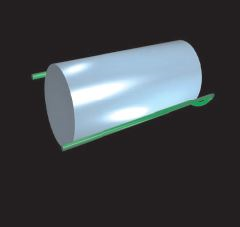Figure 2: A coil displaced 120� from the first

flow in these coils when current flows out of the page in a, b, c conductors and into a', b' and c' respectively.

If we look at the voltage induced in these coils as phasors, the diagram looks as shown in fig. 5. The main flux is taken as the reference phasor. Considering that the induced emf is —dψ/dt where ψ is the flux linkage, the diagram is drawn as shown.

As usual, the horizontal component of these phasors gives the instantaneous values of the induced emf in these coils.

Let these coils be purely resistive. Then these emf phasors also represent the currents flowing in these coils. If we consider the instant t = 0, it can be seen that

1. The field flux is along 0� axis.
2. The current in a phase coil is zero.
3. The current in b phase coil is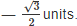4. The current in c phase coil is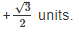These currents act to produce mmf and flux along the axes of the respective coils. Let us consider the space around b' and c coil sides. The situation is shown in fig. 6.

The resulting flux pattern causes a tendency to move in the anticlockwise direction. This is easy to see through the so called whiplash rule. Alternatively, since the force on a current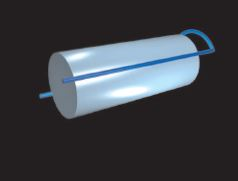Figure 3: A coil displaced 240 from the ﬁrst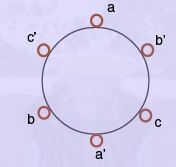Figure 4: Coils on the rotor

carrying conductor is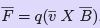, it can be seen that the torque produced tends to rotate the rotor counter-clockwise. The magnitude of the torque would increase with the current magnitude in the coils. This current is in turn dependent on the magnitude of the main field flux and its speed of rotation. Therefore one may say that motion of the main field tends to drag the rotor along with it.

When the rotor is free to move and begins moving, the motion reduces the relative speed between the main field and the rotor coils. Less emf would therefore be induced and the torque would come down. Depending on the torque requirement for the load, the difference in speed between the rotor and the main field settles down at some particular value.

From the foregoing, the following may be noted.

1. The torque produced depends on a non-zero relative speed between the ﬁeld and the rotor.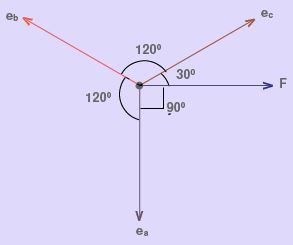Figure 5: EMF induced in the coils : Resistive rotor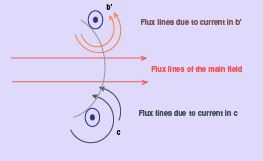Figure 6: Flux around conductors : Resistive rotor

2. It is therefore not possible for the rotor to run continuously at the same speed of the ﬁeld. This is so because in such a condition, no emf would be induced in the rotor and hence no rotor current, no torque.

3. The frequency of currents induced in the rotor coils and their magnitude depends on this diﬀerence in speed.

These are important conclusions. The speed of the main field is known as the synchronous speed, ns. If the actual speed of the rotor is nr then the ratio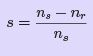(5)

is known as slip and is frequently expressed as a percentage. Typically induction machines

are designed to operate at about less than 4 percent slip at full load.

It is instructive to see the situation if the rotor resistance is neglected and is considered to be purely inductive. The phasor diagram of voltages and the currents would then look as shown in fig. 7.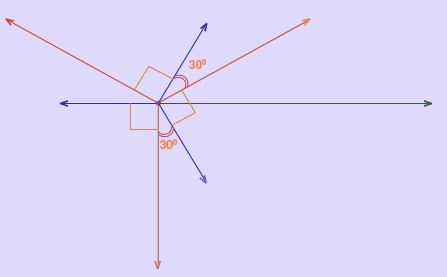Figure 7: EMF induced in coils : Inductive rotor

At t = 0, one can see that current in a phase coil is at negative maximum, while b and c phases have positive current of 0.5 units. Now if we consider the current flux profiles at coil sides a, b' , c, the picture that emerges is shown in fig. 8.

Since main flux at the a coil side is close to zero, there is very little torque produced from there. There is a tendency to move due to the b and c coil sides, but they are in opposite directions however. Hence there is no net torque on the rotor. This brings up another important conclusion — the resistance of the rotor is an important part of torque production in the induction machine. While a high resistance rotor is better suited for torque production, it would also be lossy.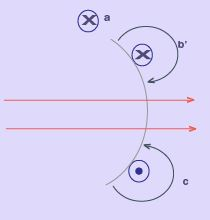Figure 8: Flux around conductors : Inductive rotor

Offer running on EduRev: Apply code STAYHOME200 to get INR 200 off on our premium plan EduRev Infinity!

## Electrical Machines for Electrical Engg.

22 videos|41 docs|26 tests

,

,

,

,

,

,

,

,

,

,

,

,

,

,

,

,

,

,

,

,

,

;